# Olympiad Test: Pattern - 1

Test Description

## 10 Questions MCQ Test Mathematics Olympiad Class 7 | Olympiad Test: Pattern - 1

Olympiad Test: Pattern - 1 for Class 7 2023 is part of Mathematics Olympiad Class 7 preparation. The Olympiad Test: Pattern - 1 questions and answers have been prepared according to the Class 7 exam syllabus.The Olympiad Test: Pattern - 1 MCQs are made for Class 7 2023 Exam. Find important definitions, questions, notes, meanings, examples, exercises, MCQs and online tests for Olympiad Test: Pattern - 1 below.
 1 Crore+ students have signed up on EduRev. Have you?
Olympiad Test: Pattern - 1 - Question 1

### Direction: Choose the appropriate number which follow the given pattern.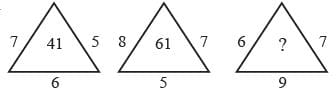Detailed Solution for Olympiad Test: Pattern - 1 - Question 1

7 ×  5 + 6 = 41
8 × 7 + 5 = 61
6 × 7 + 9 = 51

Olympiad Test: Pattern - 1 - Question 2

### Direction: Choose the appropriate number which follow the given pattern.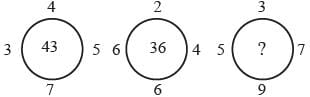Detailed Solution for Olympiad Test: Pattern - 1 - Question 2

7 × 4 + 3 × 5 = 28 + 15 = 43
6 × 2 + 6 × 4 = 12 + 24 = 36
9 × 3 + 5 × 7 = 27 + 35 = 62

Olympiad Test: Pattern - 1 - Question 3

### Direction: Choose the appropriate number which follow the given pattern.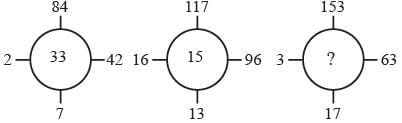Detailed Solution for Olympiad Test: Pattern - 1 - Question 3

84 ÷ 7 + 42 ÷ 2 = 12 + 21 = 33
117 ÷ 13 + 96 ÷ 16 = 9 + 6 = 15
153 ÷ 17 + 63 ÷ 3 = 9 + 21 = 30

Olympiad Test: Pattern - 1 - Question 4

Direction: Choose the appropriate number which follow the given pattern.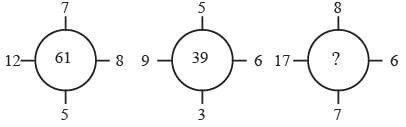Detailed Solution for Olympiad Test: Pattern - 1 - Question 4

12 × 8 – 7 × 5 = 96 – 35 = 61
9 × 6 – 5 × 3 = 54 – 15 = 39
17 × 6 – 8 × 7 = 102 – 56 = 46

Olympiad Test: Pattern - 1 - Question 5

Direction: Choose the appropriate number which follow the given pattern.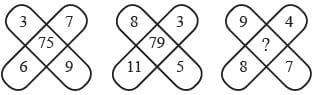Detailed Solution for Olympiad Test: Pattern - 1 - Question 5

3 × 7 + 6 × 9 = 21 + 54 = 75
8 × 3 + 11 × 5 = 24 + 55 = 79
9 × 4 + 8 × 7 = 36 + 56 = 92

Olympiad Test: Pattern - 1 - Question 6

Direction: Choose the appropriate number which follow the given pattern.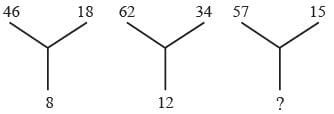Detailed Solution for Olympiad Test: Pattern - 1 - Question 6

(46 + 18) ÷ 8 = 64 ÷ 8 = 8
(62 + 34) ÷ 8 = 96 ÷ 8 = 12
(57 + 15) ÷ 8 = 72 ÷ 8 = 9

Olympiad Test: Pattern - 1 - Question 7

Direction: Choose the appropriate number which follow the given pattern.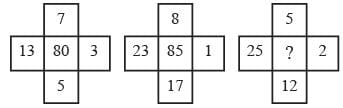Detailed Solution for Olympiad Test: Pattern - 1 - Question 7

(13 – 5)2 + (7 – 3)2 = 64 + 16 = 80
(23 – 17)2 + (8 – 1)2 = 36 + 49 = 85
(25 – 12)2 + (5 – 2)2 = 169 + 9 = 178

Olympiad Test: Pattern - 1 - Question 8

Direction: Choose the appropriate number which follow the given pattern.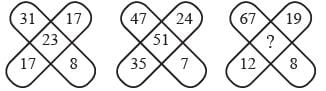Detailed Solution for Olympiad Test: Pattern - 1 - Question 8

(31 + 17) – (17 + 8) = 48 – 25 = 23
(47 + 35) – (24 + 7) = 82 – 31 = 51
(67 + 12) – (19 + 8) = 79 – 27 = 52

Olympiad Test: Pattern - 1 - Question 9

Direction: Choose the appropriate number which follow the given pattern.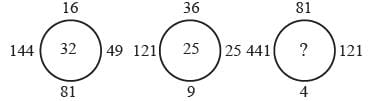Detailed Solution for Olympiad Test: Pattern - 1 - Question 9

√16 + √144 + √81+ √49 = 4 + 12 + 9 + 7
= 32
√36 + √121 + √9+ √25  = 6 + 11 + 3 + 5
= 25
√81 + √441 + √4+ √121 = 9 + 21 + 2 + 11
= 43

Olympiad Test: Pattern - 1 - Question 10

Direction: Choose the appropriate number which follow the given pattern.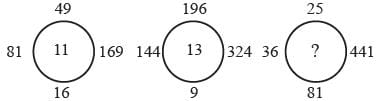Detailed Solution for Olympiad Test: Pattern - 1 - Question 10

(√81 + √169 ) − ( √49+ √16 )
= (9 + 13) – (7 + 4) = 11
(√144 + √324 ) − ( √196 + √9 )
= (12 + 18) – (14 + 3) = 13
(√36 + √441) − ( √25+ √81)
= (6 + 21) – (5 + 9) = 13